Home Blog Display Formatted Math Equations in WordPress with Mathjax and Shortcode

# Display Formatted Math Equations in WordPress with Mathjax and Shortcode

0MathJax is a cross-browser (and open source) JavaScript library that displays mathematical notation in web browsers, using MathML, LaTeX and ASCIIMathML markup. MathJax downloads with web page content, scans the page content for equation markup, and typesets the math… meaning that MathJax requires no installation of software or extra fonts on the reader’s system (although you can optionally reference your own locally-hosted JS library). MathJax will run in any browser with JavaScript support, including mobile devices.

I personally used MathJax for the first time when I wrote an article for our much-neglected Flight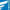website years ago (and the article remains one of a few hundred in drafts). Aviation is a very math-centric operation yet I consistently saw poor mathematical representations on aviation websites… and the less-than-inspired habit of using plain text equations certainly wasn’t good enough for us, so I wrote a quick shortcode so any of our guys could easily manufacture the excellent effect that MathJax provides.

In the last few years – and given our expertise in the area – Belief has attracted the attention of a huge number of mortgage, real-estate, and finance businesses – all of whom require nicely formatted equations from time-to-time. While we don’t use MathJax exclusively for rendering equations, it’s certainly used more often than most other solutions.

Note: Those that are in the business of finance and also have access to our platform will find a suite of APIs that will generate math equations as images. It’s part of the module that manufactures custom loan calculators.

The MathJax library is huge. For that reason, we only load the required JavaScript on pages where MathJax is used. While you may use a local install of the entire JS library, for the examples on this page we’ve chosen to use the up-to-date version of MJ made available via the MathJax Cloudflare CDN; there’s an option to alter this if you choose.

### The Result

The shortcode of $x = (-b +- sqrt(b^2-4ac))/(2a)$ will return the following formatted Math equation:

x = (-b +- sqrt(b^2-4ac))/(2a)

One of the very simple equations I used in my post on Flight was used to provide a rough altitude correction for flying in cold weather conditions (relevant for circling or other non-precision approaches). The shortcode of $text{Correction} (feet) = 4 times DeltaISA times text{altitude (feet)}/1000$ returns:

text{Correction} (feet) = 4 times DeltaISA times text{altitude (feet)}/1000

### MathJax Options

While this shortcode will work “out-of-the-box”, there’s a huge number of options available, so it’s worth visiting the MathJax website and acquainting yourself with the plethora of available configurations. By default we’ll use a MathJax configuration for AM_HTMLorMML that “… allows math to be specified using AsciiMath notation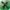, producing output in MathML if the browser supports it sufficiently, or as HTML-with-CSS otherwise.” It’s worth reading the getting startedliterature so you can decide on what flavour of notation you choose to use. You can read up on AsciiMath notation here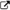.

MathML has currently become an official HTML5 recommendation. That said, it’s expected that AsciiMath will continue to be supported.

Because we only load the library on pages only when it’s necessary, we load the full version (by appending -full to the JS configuration file request URL) since it includes the complete HTML-CSS output processor (we use AM_HTMLorMML-full instead of AM_HTMLorMML).

We plan on writing a plugin that deals with some of the complexities of the plugin, and some of the options available beyond the basic implementation.

### The Code

Copy and paste the WordPress function into your theme's functions.php file or, if you sensibly have one installed, your custom functions plugin.

If you require shortcode to work in a sidebar widget, you'll have to enable the functionality with a filter. If you're using our custom functions plugin, you'll have that feature enabled by default.

The only attribute currently available is size. Alter with math size=”2″equation-in-here/math. Our updated code (or plugin) will be more comprehensive.

• MathJaxhome page.
• Download self hosted MathJax on Github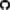.
• Install your own hosted MathJax.
• Getting Startedwith MathJax.
• Using Configuration files.
• AsciiMath.org– notation table.
• MathJax AsciiMath Support• MathJax tutorial on StackExchange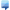(deals with TeX-AMS-MML_HTMLorMML library).
• Try a live demo on MathJax.org.
• Original MathJax homepage with lots of notation examples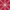.
• MathML on Wikipedia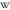.

### MathJax AsciiMath Examples

The following are just a few exmaples to demonstrate AsciiMath renderings. Because they’re rendered into MathML you’ll be able to copy them into Microsoft Word, Open Office, and other applications.

$sqrt(2)/2$ returns:

sqrt(2)/2

$(pi)r^2 or pi*r^2$ returns:

(pi)r^2

$((A+X)/2 , (B+Y)/2)$ returns:

((A+X)/2 , (B+Y)/2)

$~np~f'(x) = lim_(Deltax->0)(f(x+Deltax)-f(x))/(Deltax)$ returns:

~np~f'(x) = lim_(Deltax->0)(f(x+Deltax)-f(x))/(Deltax)

$x = (-b+-sqrt(b^2-4ac))/(2a)$ returns:

x = (-b+-sqrt(b^2-4ac))/(2a)

$d/dx[int_a^x f(t) dt] = f(x)$ returns:

d/dx[int_a^x f(t) dt] = f(x)

$m = (y_2 – y_1)/(x_2 – x_1) = (Deltay)/(Deltax)$ returns:

m = (y_2 – y_1)/(x_2 – x_1) = (Deltay)/(Deltax)

$d/dx [x^n] = nx^(n – 1)$ returns:

d/dx [x^n] = nx^(n – 1)

### Considerations

• The shortcode we’ve written is basic and doesn’t come close to embracing the full set of features. If there’s something you want added, let us know. We’ve included only what’s necessary for basic usage that suit most users.
• The shortcode attribute of size is available so the equations are appropriately scaled.
• The MathJax CDN shut down on April 30, 2017 (they’re currently providing a redirect). If you were using an earlier version of our code, you should at a minimum alter the MathJax library location.